1. 颜色量化算法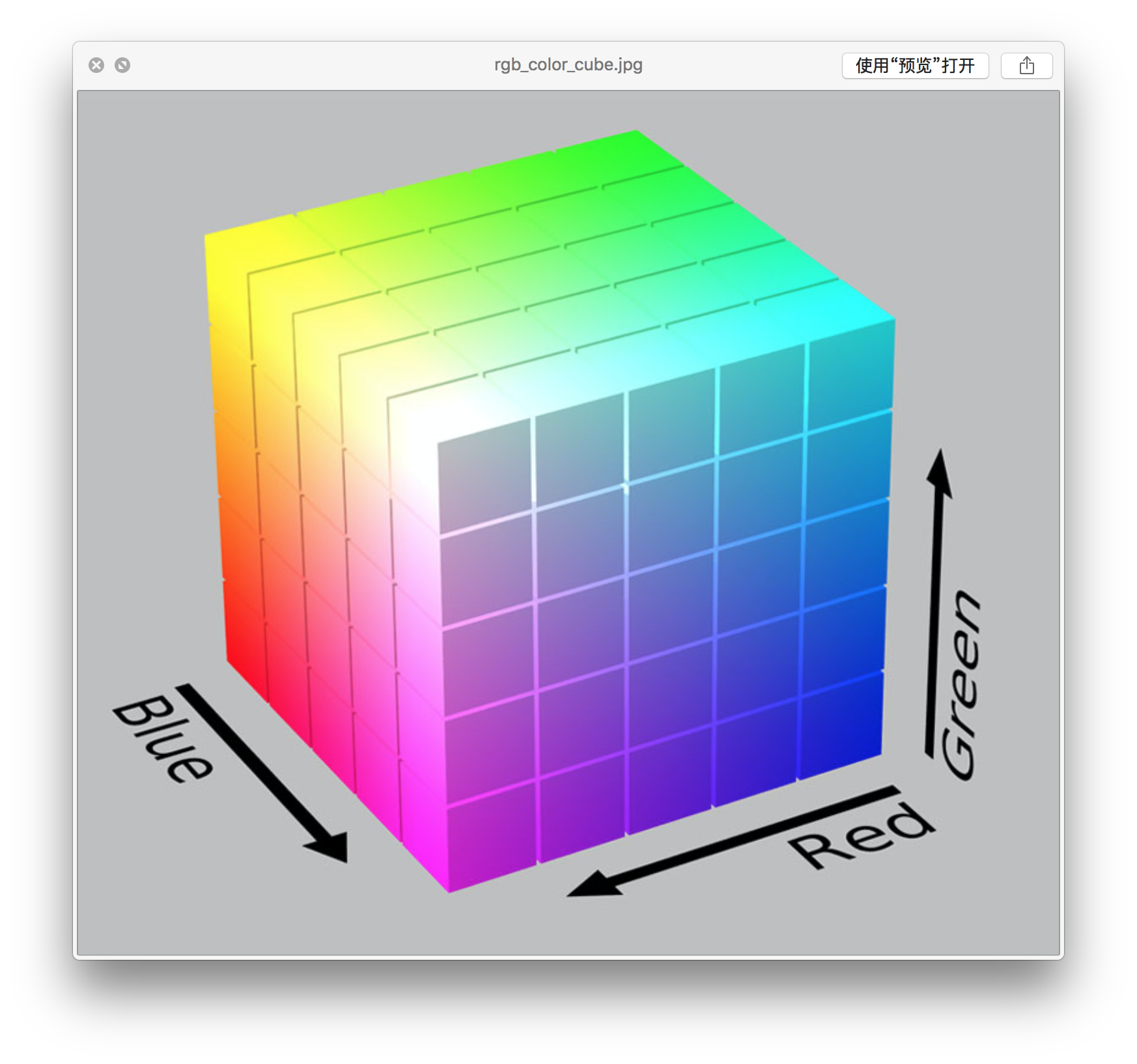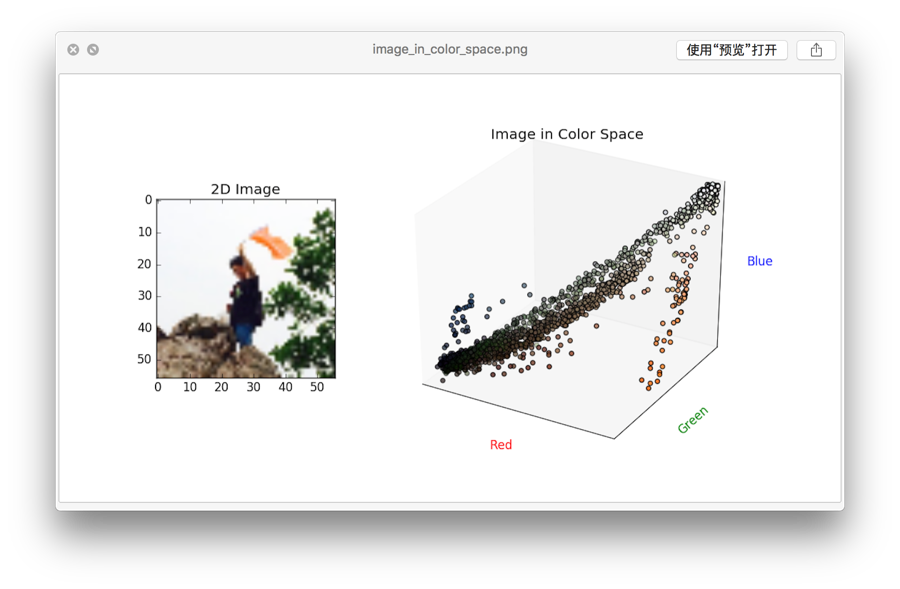1.1. 中位切分法（Median cut）

GitHub: color-theif 项目采用了 Leptonica 中的用到的（调整）中位切分法，Js 代码比 C 要易读得多。中位切分算法的原理很简单直接，将图像颜色看作是色彩空间中的长方体（VBox），从初始整个图像作为一个长方体开始，将RGB中最长的一边从颜色统计的中位数一切为二，使得到的两个长方体所包含的像素数量相同，重复上述步骤，直到最终切分得到长方体的数量等于主题颜色数量为止。

Leptonica 作者在报告 Median-Cut Color Quantization 中总结了这一算法存在的一些问题，其中主要问题是有可能存在某些条件下 VBox 体积很大但只包含少量像素。解决的方法是，每次进行切分时，并不是对上一次切分得到的所有VBox进行切分，而是通过一个优先级队列进行排序，刚开始时这一队列以VBox仅以VBox所包含的像素数作为优先级考量，当切分次数变多之后，将体积*包含像素数作为优先级。

Python 3 中内置了PriorityQueue：

from queue import PriorityQueue as PQueue

class VBox(object):
def __init__(self, r1, r2, g1, g2, b1, b2, histo):
self.vol = calV()
self.npixs = calN()
self.priority = self.npixs * -1 # PQueue 是按优先级自小到大排序

boxQueue.put((vbox0.priority, vbox0))

vbox.priority *= vbox.vol
boxQueue.put((vbox0.priority, vbox0))  

def getColorIndex(self, r, g, b):
return (r << (2 * self.SIGBITS)) + (g << self.SIGBITS) + b
def getPixHisto(self):
pixHisto = np.zeros(1 << (3 * self.SIGBITS))
for y in range(self.h):
for x in range(self.w):
r = self.pixData[y, x, 0] >> self.rshift
g = self.pixData[y, x, 1] >> self.rshift
b = self.pixData[y, x, 2] >> self.rshift

pixHisto[self.getColorIndex(r, g, b)] += 1
return pixHisto

def testMMCQ(pixDatas, maxColor):
start  = time.process_time()
themes = list(map(lambda d: MMCQ(d, maxColor).quantize(), pixDatas))
print("MMCQ Time cost: {0}".format(time.process_time() - start))
return themes
imgs = map(lambda i: 'imgs/photo%s.jpg' % i, range(1,5))
pixDatas = list(map(getPixData, imgs))
maxColor = 7

themes = [testMMCQ(pixDatas, maxColor)]
imgPalette(pixDatas, themes, ["MMCQ Palette"])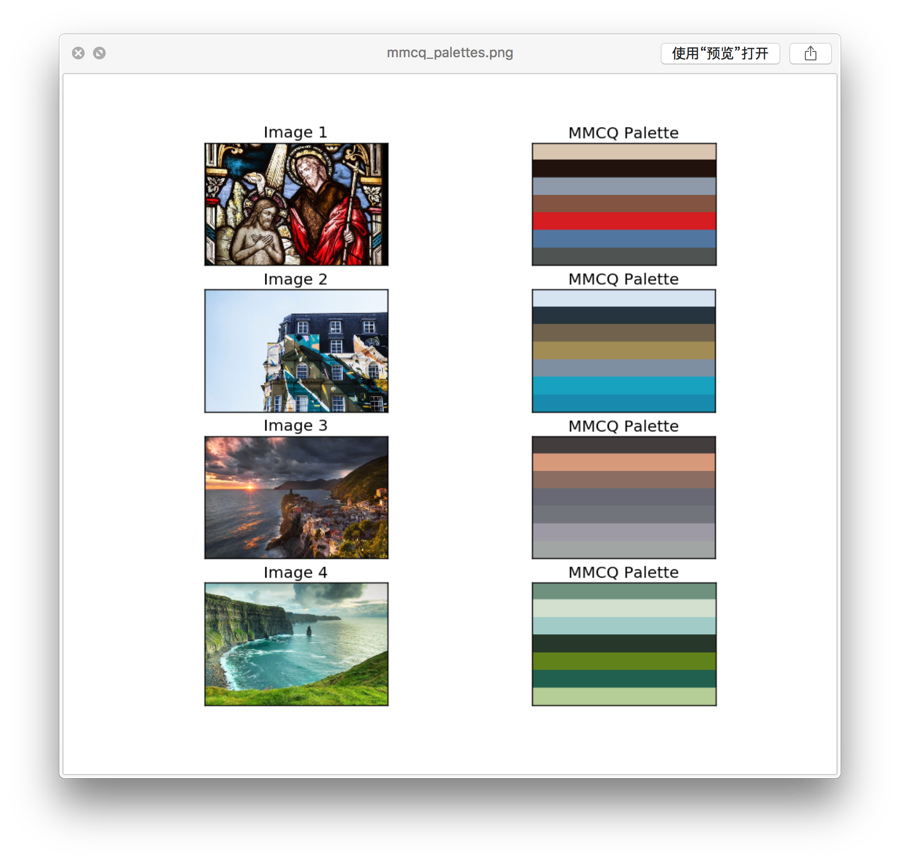1.2. 八叉树算法（Octree）

def getColors(self, node):
if node.isLeaf:
[r, g, b] = list(map(lambda n: int(n / n), zip([node.r, node.g, node.b], [node.n]*3)))
self.theme.append([r,g,b, node.n])
else:
for i in range(8):
if node.children[i] is not None:
self.getColors(node.children[i])
self.theme = sorted(self.theme, key=lambda c: -1*c)
return list(map(lambda l: l[:-1],self.theme[:self.maxColor]))  

def testOQ(pixDatas, maxColor):
start  = time.process_time()
themes = list(map(lambda d: OQ(d, maxColor).quantize(), pixDatas))
print("OQ Time cost: {0}".format(time.process_time() - start))
return themes
themes = [testMMCQ(pixDatas, maxColor), testOQ(pixDatas, maxColor)]
imgPalette(pixDatas, themes, ["MMCQ Palette", "OQ Palette"])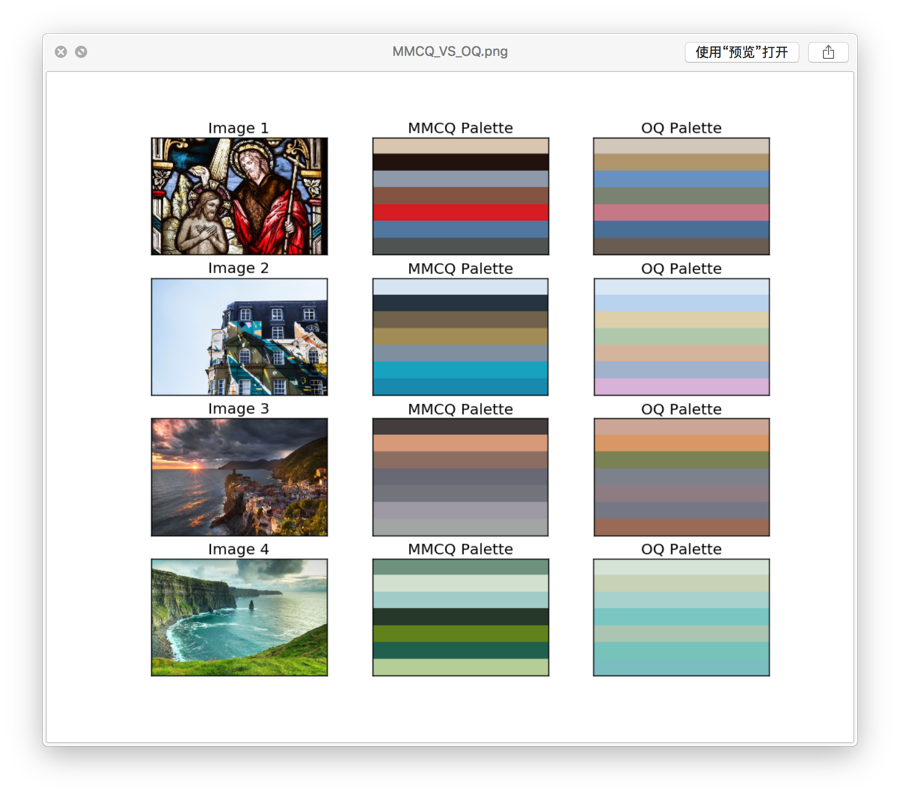#MMCQ Time cost: 8.238793
#OQ Time cost: 55.173573

2. 聚类

KMeans算法

KMeans算法的原理更加简洁：“物以类聚”。我们目的是将一堆零散的数据（如上面图2）归为k个类别，使得每个类别中的每个数据样本，距离该类别的中心（质心，centroid）距离最小，数学公式为：

Ni=0minμjC(||xiμj||2)$∑_{i=0}^{N}min_{μ_{j}∈C}(||x_{i}−μ_{j}||^{2})$

from sklearn.cluster import KMeans as KM
import numpy as np

#@pixData      image pixels stored in numpy.ndarray
#@maxColor     theme color number
h, w, d = pixData.shape
data = np.reshape((h*w, d))
km = KM(n_clusters=maxColor)
km.fit(data)
theme = np.array(km.cluster_centers_, dtype=np.uint8)    
imgs = map(lambda i: 'imgs/photo%s.jpg' % i, range(1,5))
pixDatas = list(map(getPixData, imgs))
maxColor = 7
themes = [testKmeans(pixDatas, maxColor), testKmeans(pixDatas, maxColor, useSklearn=False)]
imgPalette(pixDatas, themes, ["KMeans Palette", "KMeans DIY"])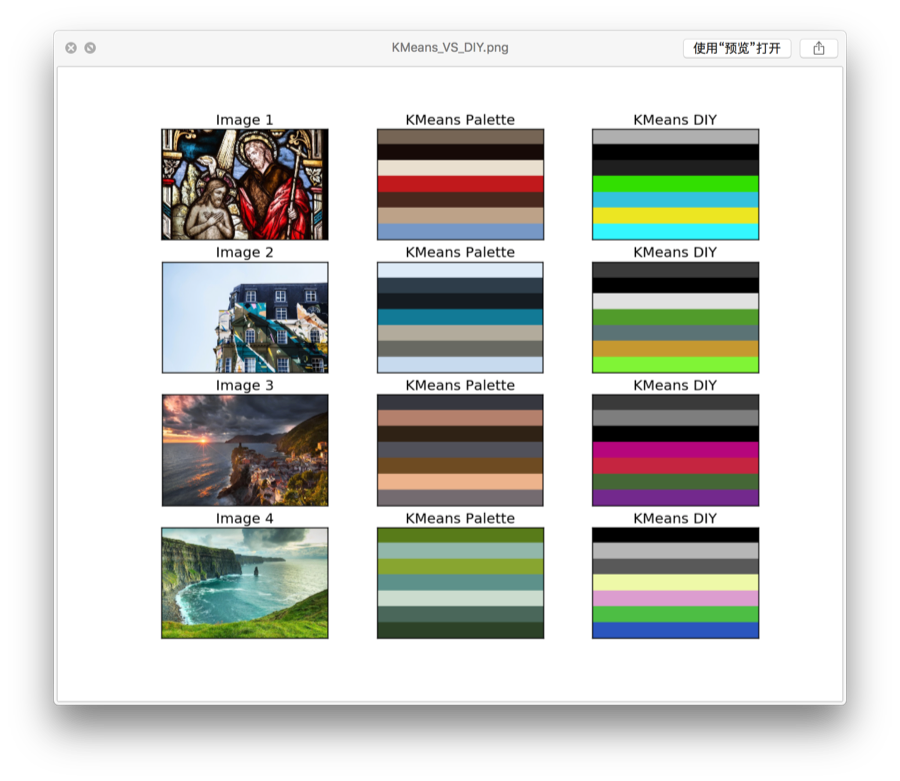3. 色彩建模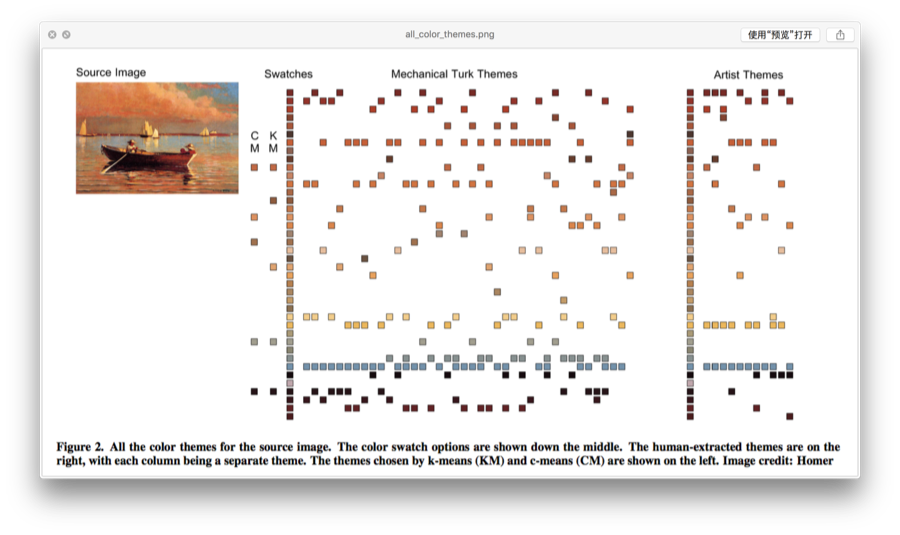79个特征的多元回归模型，不知道会不会出现过度拟合？另外虽然比前面算法多了很多特征，但仍旧多物理特征。对人类观察者来说，我们看到的并非一堆无意义的色块，虽然有研究表明颜色信息并非场景识别的必要线索，但反过来场景图像中的语义信息却很有可能影响颜色对观察者的意义，这大概就是心理学研究与计算机科学方向上的差异。

总结

if(post.content.isHelpful){
\$("button#donate").click();
};